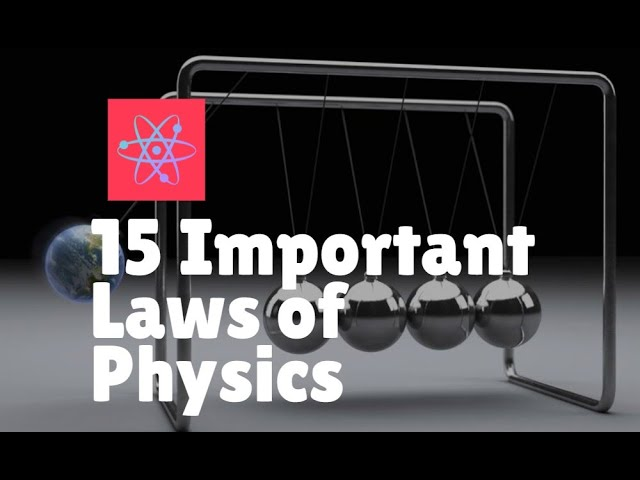# Key Laws of Physics: 15 Essential Principles ExplainedTLDR Physics laws are fundamental conclusions drawn from scientific observations and experiments, and they describe various phenomena and principles in the natural world.

## Key insights

• 🌊
Archimedes' principle states that a body immersed in a fluid experiences an upward thrust equal to the weight of the fluid displaced by it, which can be controversial in certain contexts.
• 🚀
Newton's first law of motion states that an object will continue in its current state of motion unless acted upon by an external force.
• 🔫
Newton's third law of motion explains the recoil felt on pulling the trigger of a gun.
• 🌊
Bernoulli's principle states that as the speed of a moving fluid increases, the pressure within the fluid decreases, which explains the aerodynamic lift on the wing of an airplane.
• 🔥
The law of conservation of energy states that energy cannot be created or destroyed, only transformed from one form to another, which challenges the idea of perpetual motion machines.

## Timestamped Summary

• 📚
00:00
Physics laws are fundamental conclusions drawn from scientific observations and experiments, and some important ones include Archimedes' principle.
• 💡
00:43
When a body is immersed in a fluid, it experiences an upward thrust equal to the weight of the fluid displaced; Avogadro's law states that equal volumes of gases contain equal numbers of molecules under the same conditions; Ohm's law states that current is directly proportional to potential difference in a conductor; Newton's laws describe the law of gravitation.
• 🌍
01:27
Objects attract each other based on their masses and distance, causing objects to fall towards the earth, while Newton's first law states that objects will remain at rest or in motion unless acted upon by external forces.
• 📚
02:02
Newton's second law states that force is equal to mass multiplied by acceleration, while Newton's third law states that for every action there is an equal and opposite reaction, and Newton's law of cooling states that the rate at which a body cools is proportional to its excessive mean temperature.
• 💡
02:43
The force between two electric charges decreases by a quarter when the distance between them is doubled, and the total energy radiated from a blackbody is equal to the fourth power of its absolute temperature.
• 💡
03:23
Pressure change is transmitted through fluids without loss, hydraulic machines work on this principle, atmospheric pressure decreases with height, Hookes law states that spring extension is proportional to tension, and Bernoulli's principle explains the decrease in pressure as fluid speed increases.
• 🔬
04:14
Temperature of a gas varies inversely with its pressure, volume of a gas changes with temperature, and planets orbit the Sun in elliptical paths.
• 💡
04:57
The orbital periods of planets are related to their distance from the Sun, energy is conserved in the universe, the Tyndall effect scatters light, and the rate of diffusion of gases is inversely proportional to their density.Report the articleThank you for the feedback